### 2.1 SVM思想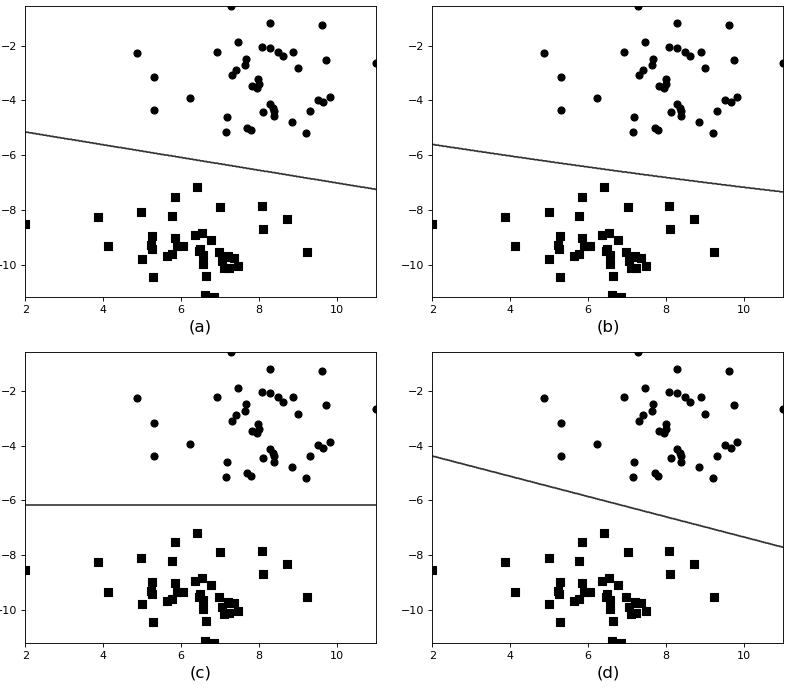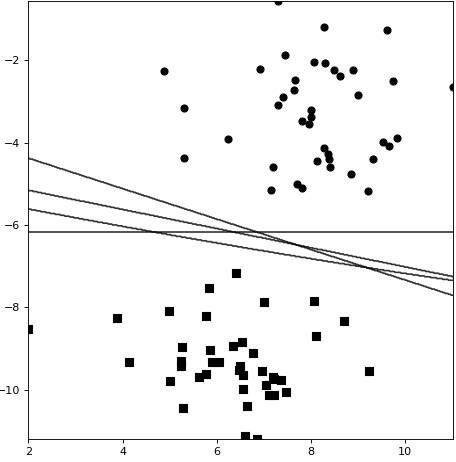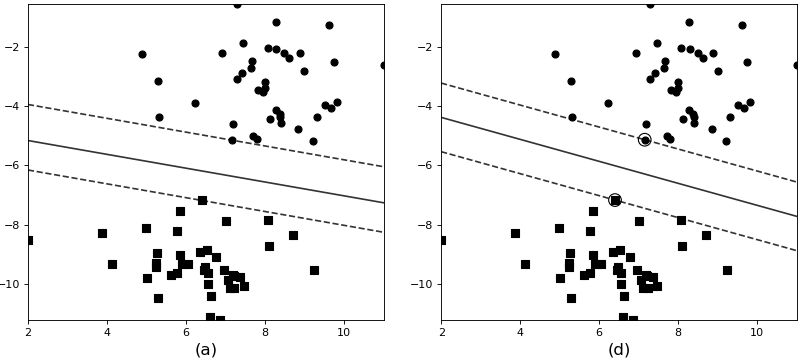### 2.2 SVM原理

#### 2.2.1超平面的表达

${w}^{T}x+b=0\phantom{\rule{0.278em}{0ex}}\phantom{\rule{0.278em}{0ex}}\phantom{\rule{0.278em}{0ex}}\phantom{\rule{0.278em}{0ex}}\phantom{\rule{0.278em}{0ex}}\phantom{\rule{0.278em}{0ex}}\phantom{\rule{0.278em}{0ex}}\phantom{\rule{0.278em}{0ex}}\phantom{\rule{0.278em}{0ex}}\phantom{\rule{0.278em}{0ex}}\left(1\right)$

#### 2.2.2函数间隔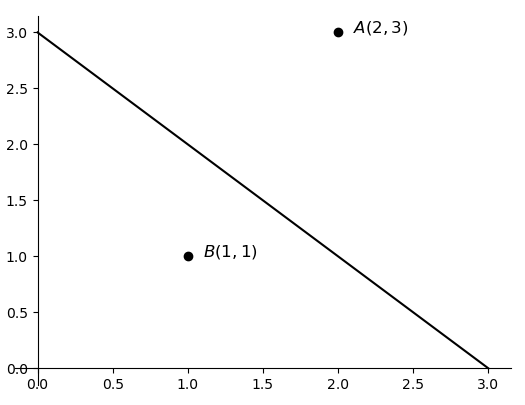${\stackrel{^}{\gamma }}^{\left(i\right)}={y}^{\left(i\right)}\left({w}^{T}{x}^{\left(i\right)}+b\right)\phantom{\rule{0.278em}{0ex}}\phantom{\rule{0.278em}{0ex}}\phantom{\rule{0.278em}{0ex}}\phantom{\rule{0.278em}{0ex}}\phantom{\rule{0.278em}{0ex}}\phantom{\rule{0.278em}{0ex}}\phantom{\rule{0.278em}{0ex}}\phantom{\rule{0.278em}{0ex}}\phantom{\rule{0.278em}{0ex}}\phantom{\rule{0.278em}{0ex}}\left(2\right)$

$\stackrel{^}{\gamma }=\underset{i=1,2,...,m}{min}\phantom{\rule{0.167em}{0ex}}{\stackrel{^}{\gamma }}^{\left(i\right)}\phantom{\rule{0.278em}{0ex}}\phantom{\rule{0.278em}{0ex}}\phantom{\rule{0.278em}{0ex}}\phantom{\rule{0.278em}{0ex}}\phantom{\rule{0.278em}{0ex}}\phantom{\rule{0.278em}{0ex}}\phantom{\rule{0.278em}{0ex}}\phantom{\rule{0.278em}{0ex}}\phantom{\rule{0.278em}{0ex}}\phantom{\rule{0.278em}{0ex}}\left(3\right)$

#### 2.2.3 几何间隔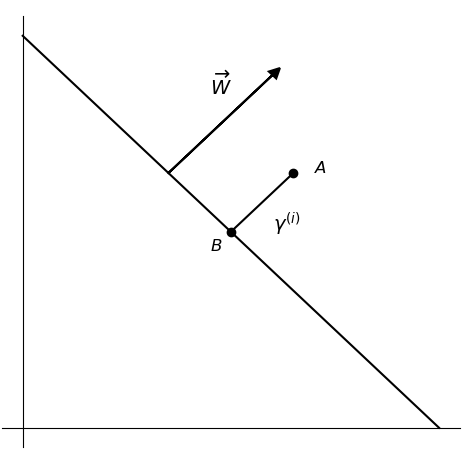${x}^{\left(i\right)}-{\gamma }^{\left(i\right)}\cdot \frac{W}{||W||}\phantom{\rule{0.278em}{0ex}}\phantom{\rule{0.278em}{0ex}}\phantom{\rule{0.278em}{0ex}}\phantom{\rule{0.278em}{0ex}}\phantom{\rule{0.278em}{0ex}}\phantom{\rule{0.278em}{0ex}}\phantom{\rule{0.278em}{0ex}}\phantom{\rule{0.278em}{0ex}}\phantom{\rule{0.278em}{0ex}}\phantom{\rule{0.278em}{0ex}}\left(4\right)$

${w}^{T}\left({x}^{\left(i\right)}-{\gamma }^{\left(i\right)}\cdot \frac{W}{||W||}\right)+b=0\phantom{\rule{0.278em}{0ex}}\phantom{\rule{0.278em}{0ex}}\phantom{\rule{0.278em}{0ex}}\phantom{\rule{0.278em}{0ex}}\phantom{\rule{0.278em}{0ex}}\phantom{\rule{0.278em}{0ex}}\phantom{\rule{0.278em}{0ex}}\phantom{\rule{0.278em}{0ex}}\phantom{\rule{0.278em}{0ex}}\phantom{\rule{0.278em}{0ex}}\left(5\right)$

${w}^{T}\left({x}^{\left(i\right)}-{\gamma }^{\left(i\right)}\cdot \frac{w}{||w||}\right)+b=0\phantom{\rule{0.278em}{0ex}}\phantom{\rule{0.278em}{0ex}}\phantom{\rule{0.278em}{0ex}}\phantom{\rule{0.278em}{0ex}}\phantom{\rule{0.278em}{0ex}}\phantom{\rule{0.278em}{0ex}}\phantom{\rule{0.278em}{0ex}}\phantom{\rule{0.278em}{0ex}}\phantom{\rule{0.278em}{0ex}}\phantom{\rule{0.278em}{0ex}}\left(6\right)$

${\gamma }^{\left(i\right)}=\frac{{w}^{T}{x}^{\left(i\right)}+b}{||w||}={\left(\frac{w}{||w||}\right)}^{T}{x}^{\left(i\right)}+\frac{b}{||w||}\phantom{\rule{0.278em}{0ex}}\phantom{\rule{0.278em}{0ex}}\phantom{\rule{0.278em}{0ex}}\phantom{\rule{0.278em}{0ex}}\phantom{\rule{0.278em}{0ex}}\phantom{\rule{0.278em}{0ex}}\phantom{\rule{0.278em}{0ex}}\phantom{\rule{0.278em}{0ex}}\phantom{\rule{0.278em}{0ex}}\phantom{\rule{0.278em}{0ex}}\left(7\right)$

${\gamma }^{\left(i\right)}={y}^{\left(i\right)}\left({\left(\frac{w}{||w||}\right)}^{T}{x}^{\left(i\right)}+\frac{b}{||w||}\right)\phantom{\rule{0.278em}{0ex}}\phantom{\rule{0.278em}{0ex}}\phantom{\rule{0.278em}{0ex}}\phantom{\rule{0.278em}{0ex}}\phantom{\rule{0.278em}{0ex}}\phantom{\rule{0.278em}{0ex}}\phantom{\rule{0.278em}{0ex}}\phantom{\rule{0.278em}{0ex}}\phantom{\rule{0.278em}{0ex}}\phantom{\rule{0.278em}{0ex}}\left(8\right)$

$\begin{array}{rl}& {\gamma }^{A}=+1\cdot \left({\left(\frac{w}{||w||}\right)}^{T}{x}^{\left(A\right)}+\frac{b}{||w||}\right)={\left(\frac{\left(1,1\right)}{\sqrt{1+1}}\right)}^{T}\left(2,3\right)+\frac{-3}{\sqrt{1+1}}=\sqrt{2}\\ & {\gamma }^{B}=-1\cdot \left({\left(\frac{w}{||w||}\right)}^{T}{x}^{\left(A\right)}+\frac{b}{||w||}\right)=-{\left(\frac{\left(1,1\right)}{\sqrt{1+1}}\right)}^{T}\left(1,1\right)+\frac{3}{\sqrt{1+1}}=\frac{1}{\sqrt{2}}\end{array}\phantom{\rule{0.278em}{0ex}}\phantom{\rule{0.278em}{0ex}}\phantom{\rule{0.278em}{0ex}}\phantom{\rule{0.278em}{0ex}}\phantom{\rule{0.278em}{0ex}}\phantom{\rule{0.278em}{0ex}}\phantom{\rule{0.278em}{0ex}}\phantom{\rule{0.278em}{0ex}}\phantom{\rule{0.278em}{0ex}}\phantom{\rule{0.278em}{0ex}}\left(9\right)$

$\gamma =\underset{i=1,2,...,m}{min}\phantom{\rule{0.167em}{0ex}}{\gamma }^{\left(i\right)}\phantom{\rule{0.278em}{0ex}}\phantom{\rule{0.278em}{0ex}}\phantom{\rule{0.278em}{0ex}}\phantom{\rule{0.278em}{0ex}}\phantom{\rule{0.278em}{0ex}}\phantom{\rule{0.278em}{0ex}}\phantom{\rule{0.278em}{0ex}}\phantom{\rule{0.278em}{0ex}}\phantom{\rule{0.278em}{0ex}}\phantom{\rule{0.278em}{0ex}}\left(10\right)$

$\gamma =\frac{\stackrel{^}{\gamma }}{||w||}\phantom{\rule{0.278em}{0ex}}\phantom{\rule{0.278em}{0ex}}\phantom{\rule{0.278em}{0ex}}\phantom{\rule{0.278em}{0ex}}\phantom{\rule{0.278em}{0ex}}\phantom{\rule{0.278em}{0ex}}\phantom{\rule{0.278em}{0ex}}\phantom{\rule{0.278em}{0ex}}\phantom{\rule{0.278em}{0ex}}\phantom{\rule{0.278em}{0ex}}\left(11\right)$

#### 2.2.4 最大间隔分类器

$\gamma$$\gamma$尽可能大，因为目的就是最大化$\gamma$$\gamma$;

② 同时要使得样本中所有的几何距离都大于$\gamma$$\gamma$，因为由式$\left(10\right)$$(10)$可知$\gamma$$\gamma$是所有间隔中的最小值；

#### 2.2.5 函数间隔的性质

$\stackrel{^}{\gamma }={y}^{\left(i\right)}\left({w}^{T}{x}^{\left(i\right)}+b\right)\phantom{\rule{0.278em}{0ex}}\phantom{\rule{0.278em}{0ex}}\phantom{\rule{0.278em}{0ex}}\phantom{\rule{0.278em}{0ex}}\phantom{\rule{0.278em}{0ex}}\phantom{\rule{0.278em}{0ex}}\phantom{\rule{0.278em}{0ex}}\phantom{\rule{0.278em}{0ex}}\phantom{\rule{0.278em}{0ex}}\phantom{\rule{0.278em}{0ex}}\left(16\right)$

${y}^{\left(i\right)}\left({\left(\frac{w}{\stackrel{^}{\gamma }}\right)}^{T}{x}^{\left(i\right)}+\frac{b}{\stackrel{^}{\gamma }}\right)=1\phantom{\rule{0.278em}{0ex}}\phantom{\rule{0.278em}{0ex}}\phantom{\rule{0.278em}{0ex}}\phantom{\rule{0.278em}{0ex}}\phantom{\rule{0.278em}{0ex}}\phantom{\rule{0.278em}{0ex}}\phantom{\rule{0.278em}{0ex}}\phantom{\rule{0.278em}{0ex}}\phantom{\rule{0.278em}{0ex}}\phantom{\rule{0.278em}{0ex}}\left(17\right)$

${y}^{\left(i\right)}\left({W}^{T}{x}^{\left(i\right)}+B\right)=1\phantom{\rule{0.278em}{0ex}}\phantom{\rule{0.278em}{0ex}}\phantom{\rule{0.278em}{0ex}}\phantom{\rule{0.278em}{0ex}}\phantom{\rule{0.278em}{0ex}}\phantom{\rule{0.278em}{0ex}}\phantom{\rule{0.278em}{0ex}}\phantom{\rule{0.278em}{0ex}}\phantom{\rule{0.278em}{0ex}}\phantom{\rule{0.278em}{0ex}}\left(18\right)$

${y}^{\left(i\right)}\left({w}^{T}{x}^{\left(i\right)}+b\right)=1\phantom{\rule{0.278em}{0ex}}\phantom{\rule{0.278em}{0ex}}\phantom{\rule{0.278em}{0ex}}\phantom{\rule{0.278em}{0ex}}\phantom{\rule{0.278em}{0ex}}\phantom{\rule{0.278em}{0ex}}\phantom{\rule{0.278em}{0ex}}\phantom{\rule{0.278em}{0ex}}\phantom{\rule{0.278em}{0ex}}\phantom{\rule{0.278em}{0ex}}\left(19\right)$

$2{x}_{1}+4{x}_{2}-8=0\phantom{\rule{0.278em}{0ex}}\phantom{\rule{0.278em}{0ex}}\phantom{\rule{0.278em}{0ex}}\phantom{\rule{0.278em}{0ex}}\phantom{\rule{0.278em}{0ex}}\phantom{\rule{0.278em}{0ex}}\phantom{\rule{0.278em}{0ex}}\phantom{\rule{0.278em}{0ex}}\phantom{\rule{0.278em}{0ex}}\phantom{\rule{0.278em}{0ex}}\left(20\right)$

$+1\left(2{x}_{1}^{\left(k\right)}+4{x}_{2}^{\left(k\right)}-8\right)=2\phantom{\rule{0.278em}{0ex}}\phantom{\rule{0.278em}{0ex}}\phantom{\rule{0.278em}{0ex}}\phantom{\rule{0.278em}{0ex}}\phantom{\rule{0.278em}{0ex}}\phantom{\rule{0.278em}{0ex}}\phantom{\rule{0.278em}{0ex}}\phantom{\rule{0.278em}{0ex}}\phantom{\rule{0.278em}{0ex}}\phantom{\rule{0.278em}{0ex}}\left(21\right)$

${x}_{1}^{\left(k\right)}+2{x}_{2}^{\left(k\right)}-4=1\phantom{\rule{0.278em}{0ex}}\phantom{\rule{0.278em}{0ex}}\phantom{\rule{0.278em}{0ex}}\phantom{\rule{0.278em}{0ex}}\phantom{\rule{0.278em}{0ex}}\phantom{\rule{0.278em}{0ex}}\phantom{\rule{0.278em}{0ex}}\phantom{\rule{0.278em}{0ex}}\phantom{\rule{0.278em}{0ex}}\phantom{\rule{0.278em}{0ex}}\left(22\right)$

### 3 SVM示例代码与线性不可分

#### 3.1 线性SVM示例代码

xxxxxxxxxx711  def __init__(self,22     C=1.0, 33     kernel='rbf', 44     degree=3, 55     gamma='scale',66     coef0=0.0,77     decision_function_shape='ovr'):

xxxxxxxxxx611 def train(x_train, x_test, y_train, y_test):22     model = SVC(kernel='linear')33     model.fit(x_train, y_train)44     y_pre = model.predict(x_test)55     print(f"准确率为：{model.score(x_test, y_test)}")66     # 准确率为：0.975925925925926

#### 3.2 从线性不可分谈起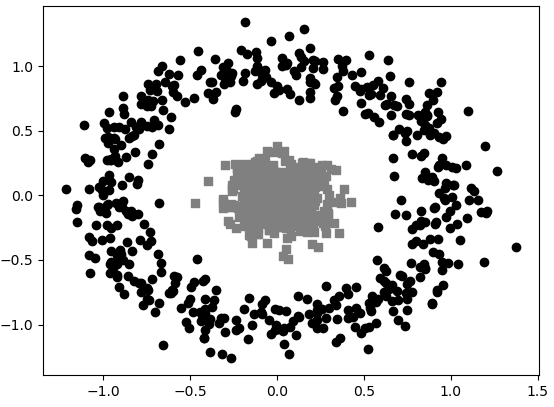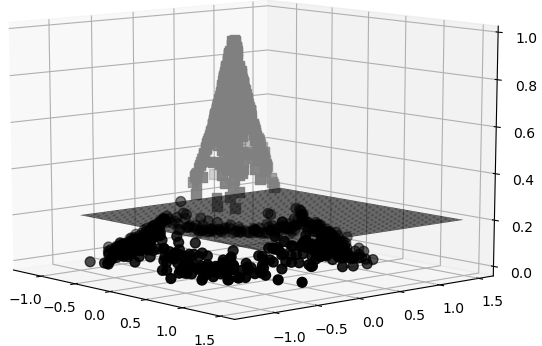#### 3.3 将低维特征映射到高维空间

$w=\sum _{i=1}^{m}{\alpha }_{i}{y}^{\left(i\right)}{x}^{\left(i\right)}\phantom{\rule{0.278em}{0ex}}\phantom{\rule{0.278em}{0ex}}\phantom{\rule{0.278em}{0ex}}\phantom{\rule{0.278em}{0ex}}\phantom{\rule{0.278em}{0ex}}\phantom{\rule{0.278em}{0ex}}\phantom{\rule{0.278em}{0ex}}\phantom{\rule{0.278em}{0ex}}\phantom{\rule{0.278em}{0ex}}\phantom{\rule{0.278em}{0ex}}\left(23\right)$

$\begin{array}{rl}& {w}^{T}x+b=\sum _{i=1}^{m}{\alpha }_{i}{y}^{\left(i\right)}{x}^{\left(i\right)}x+b\\ & =\sum _{i=1}^{m}{\alpha }_{i}{y}^{\left(i\right)}⟨{x}^{\left(i\right)},x⟩+b\end{array}\phantom{\rule{0.278em}{0ex}}\phantom{\rule{0.278em}{0ex}}\phantom{\rule{0.278em}{0ex}}\phantom{\rule{0.278em}{0ex}}\phantom{\rule{0.278em}{0ex}}\phantom{\rule{0.278em}{0ex}}\phantom{\rule{0.278em}{0ex}}\phantom{\rule{0.278em}{0ex}}\phantom{\rule{0.278em}{0ex}}\phantom{\rule{0.278em}{0ex}}\left(24\right)$

$\begin{array}{rl}& y=\sum _{i=1}^{m}{\alpha }_{i}{y}^{\left(i\right)}⟨{x}^{\left(i\right)},x⟩+b\\ & =\sum _{i=1}^{m}{\alpha }_{i}{y}^{\left(i\right)}⟨\varphi \left({x}^{\left(i\right)}\right),\varphi \left(x\right)⟩+b\end{array}\phantom{\rule{0.278em}{0ex}}\phantom{\rule{0.278em}{0ex}}\phantom{\rule{0.278em}{0ex}}\phantom{\rule{0.278em}{0ex}}\phantom{\rule{0.278em}{0ex}}\phantom{\rule{0.278em}{0ex}}\phantom{\rule{0.278em}{0ex}}\phantom{\rule{0.278em}{0ex}}\phantom{\rule{0.278em}{0ex}}\phantom{\rule{0.278em}{0ex}}\left(25\right)$

$\begin{array}{rl}& \varphi \left(x\right)={\left[{x}_{1}{x}_{1},{x}_{1}{x}_{2},{x}_{1}{x}_{3},{x}_{2}{x}_{1},{x}_{2}{x}_{2},{x}_{2}{x}_{3},{x}_{3}{x}_{1},{x}_{3}{x}_{2},{x}_{3}{x}_{3}\right]}^{T}\\ & \varphi \left(z\right)={\left[{z}_{1}{z}_{1},{z}_{1}{z}_{2},{z}_{1}{z}_{3},{z}_{2}{z}_{1},{z}_{2}{z}_{2},{z}_{2}{z}_{3},{z}_{3}{z}_{1},{z}_{3}{z}_{2},{z}_{3}{z}_{3}\right]}^{T}\\ & ⟨\varphi \left(x\right),\varphi \left(z\right)⟩=\left[{x}_{1}{x}_{1}{z}_{1}{z}_{1}+{x}_{1}{x}_{2}{z}_{1}{z}_{2}+\cdots +{x}_{3}{x}_{3}{z}_{3}{z}_{3}\right]\end{array}\phantom{\rule{0.278em}{0ex}}\phantom{\rule{0.278em}{0ex}}\phantom{\rule{0.278em}{0ex}}\phantom{\rule{0.278em}{0ex}}\phantom{\rule{0.278em}{0ex}}\phantom{\rule{0.278em}{0ex}}\phantom{\rule{0.278em}{0ex}}\phantom{\rule{0.278em}{0ex}}\phantom{\rule{0.278em}{0ex}}\phantom{\rule{0.278em}{0ex}}\left(26\right)$

#### 3.4 SVM中的核技巧

$\mathcal{X}$$\mathcal{X}$是输入空间（欧式空间${R}^{n}$$R^n$的子集或离散集合），又设$\mathcal{H}$$\mathcal{H}$为特征空间（希尔伯特空间），如果存在一个从$\mathcal{X}$$\mathcal{X}$$\mathcal{H}$$\mathcal{H}$的映射$\varphi \left(x\right):\mathcal{X}\to \mathcal{H}$$\phi (x):\mathcal{X}\to \mathcal{H}$使得对所有$x,z\in \mathcal{X}$$x,z\in\mathcal{X}$，函数$K\left(x,z\right)$$K(x,z)$满足条件$K\left(x,z\right)=\varphi \left(x\right)\cdot \varphi \left(z\right)$$K(x,z)=\phi(x)\cdot\phi(z)$，则称$K\left(x,z\right)$$K(x,z)$为核函数，$\varphi \left(x\right)$$\phi(x)$称为映射函数。